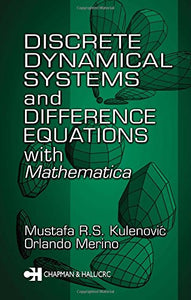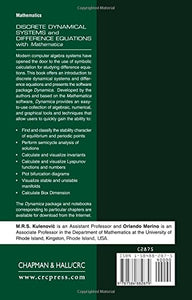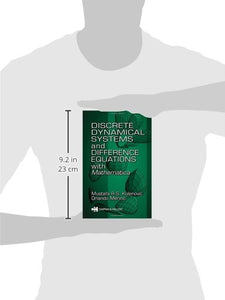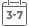# Discrete Dynamical Systems And Difference Equations With Mathematica

• Publish Date: 2002-02-27
• Binding: Hardcover
• Author: Mustafa R.S. Kulenovic;Orlando Merino
Vendor
Brand: Chapman and Hall/CRC
Regular price
\$112.87
Sale price
\$101.68
Quantity must be 1 or more

Attention: For textbook, access codes and supplements are not guaranteed with used items.Following the work of Yorke and Li in 1975, the theory of discrete dynamical systems and difference equations developed rapidly. The applications of difference equations also grew rapidly, especially with the introduction of graphical-interface software that can plot trajectories, calculate Lyapunov exponents, plot bifurcation diagrams, and find basins of attraction.

Modern computer algebra systems have opened the door to the use of symbolic calculation for studying difference equations. This book offers an introduction to discrete dynamical systems and difference equations and presents the Dynamica software. Developed by the authors and based on Mathematica, Dynamica provides an easy-to-use collection of algebraic, numerical, and graphical tools and techniques that allow users to quickly gain the ability to:

• Find and classify the stability character of equilibrium and periodic points
• Perform semicycle analysis of solutions
• Calculate and visualize invariants
• Calculate and visualize Lyapunov functions and numbers
• Plot bifurcation diagrams
• Visualize stable and unstable manifolds
• Calculate Box Dimension

While it presents the essential theoretical concepts and results, the book's emphasis is on using the software. The authors present two sets of Dynamica sessions: one that serves as a tutorial of the different techniques, the other features case studies of well-known difference equations. Dynamica and notebooks corresponding to particular chapters are available for download from the Internet.